• ## 超声波避障程序

热门讨论 2012-09-13 22:29:42
本程序是基于51单片机的超声波避障程序，实现超声波避障，广泛应用于智能小车系统中，程序中重要代码已经做了详细注释，且程序已测试通过请放心下载！
• stm32-超声波避障程序案例，循迹+壁障+遥控功能，蓝牙遥控
• 超声波避障程序 源代码 C 单片机！！！！！！！！！！！！！！！！！！！！！！！！！！
• 求一个超声波避障程序，不要显示模块，也不要外部蓝牙什么控制，简单的自动避障就行。最好是高低电平的定义来控制的 比如 void Car_Turn-Left(void) { RIN1_set; RIN2_clr; LIN1_clr; LIN2_set; 这样的就可以，谢谢 ...
求一个超声波避障程序，不要显示模块，也不要外部蓝牙什么控制，简单的自动避障就行。最好是高低电平的定义来控制的 比如 void Car_Turn-Left(void) { RIN1_set; RIN2_clr; LIN1_clr; LIN2_set; 这样的就可以，谢谢
展开全文• 能够自主检测前方距离，小于一定值时，舵机带动超声波测距传感器分别向左、向右转向，分别测距并比较，控制小车向距离较大侧转弯；如果两侧距离均小于设定值，控制小车后退，再分别检测左右距离
• C语言/程序/避障/51单片机/超声波/keil_uv2C语言
•嵌入式
• //超声波接口定义 sbit TRIG= P3^2; //超声波接口定义 sbit ENB = P2^5; //左电机高电平 sbit ENA = P2^4; //右电机高电平 sbit IN1 = P2^0; sbit IN2 = P2^1; //右电机 sbit IN3 = P2^2; sbit IN4 = ...
#include<reg52.h>
#include <intrins.h>

typedef unsigned char uchar;
typedef unsigned int  uint;
typedef unsigned long  ulong;

sbit Sevro_moto_pwm = P1^5;	   //接舵机信号端输入PWM信号调节速度
sbit  ECHO= P3^3;				   //超声波接口定义
sbit  TRIG= P3^2;				   //超声波接口定义
sbit ENB = P2^5;	  //左电机高电平
sbit ENA = P2^4;	  //右电机高电平
sbit IN1 = P2^0;
sbit IN2 = P2^1;	  //右电机
sbit IN3 = P2^2;
sbit IN4 = P2^3;	  //左电机
sbit P1_4=P1^4; 	  //LED
//sbit A1 = P1^4;		  //左红外避障模块
//sbit A2 = P2^7;		  //右红外避障模块
//sbit A3 = P1^2;		  //左红外寻迹模块
//sbit A4 = P1^3;		  //右红外寻迹模块
sbit K1 = P1^0;		  //功能转换按键
sbit K2 = P1^1;		  //加速键
sbit K3 = P1^2;	  //减速键
uchar connt;			  //调速周期
uchar PWM_time;			  //高电平时间
uchar flag1=0,flag2=0;			  //标志位
uchar COM;
uchar pwm_val_left  = 0;//变量定义
uchar push_val_left ;//舵机归中，产生约，1.5MS 信号
uchar non=0,t=0;
ulong S=0;			//距离变量定义
ulong S1=0;
ulong S2=0;
ulong S3=0;
ulong S4=0;
uint time=0;		    //时间变量
uint timer=0;			//延时基准变量

/************************************************************************/
void delay(uint k)	  //延时函数
{
uint x,y;
for(x=0;x<k;x++)
for(y=0;y<2000;y++);
}
void delay1(uint j)	  //延时函数
{
uint x,y;
for(x=0;x<j;x++)
for(y=0;y<120;y++);
}
void shansuo()
{
P1_4=1;
delay(1);
P1_4=0;
delay(1);
}
/************************************************************************/
void keyspeed(void)
{

if(K2==0)					   // 加速
{
delay1(10);
if(K2==0)
{

PWM_time--;
}
while(!K2);			   //松键检测
}
if(K3==0)					   //减速
{
delay1(10);
if(K3==0)
{

PWM_time++;
}
while(!K3);
}
}
void stop()				//刹车
{
IN1 = 0;
IN2 = 0;
IN3 = 0;
IN4 = 0;
}
void go()				//前进
{
IN1 = 1;
IN2 = 0;
IN3 = 1;
IN4 = 0;
}
void back()				//后退
{
IN1 = 0;
IN2 = 1;
IN3 = 0;
IN4 = 1;
}
void leftgo()				//左大转
{
IN1 = 0;
IN2 = 1;
IN3 = 1;
IN4 = 0;
}

void rightgo()				//右大转
{
IN1 = 1;
IN2 = 0;
IN3 = 0;
IN4 = 1;
}

/************************************************************************/
void  StartModule() 		      //启动测距信号
{
TRIG=1;
_nop_();
_nop_();
_nop_();
_nop_();
_nop_();
_nop_();
_nop_();
_nop_();
_nop_();
_nop_();
_nop_();
_nop_();
_nop_();
_nop_();
_nop_();
_nop_();
_nop_();
_nop_();
_nop_();
_nop_();
_nop_();
TRIG=0;

}

void InitUART(void)		 //串口中断初始化函数
{
SCON=0x50;			//设置为工作方式1
TMOD=0x20;			//设置计数器工作方式2
PCON=0x00;			//波特率不加倍
TH1=0xfd;				//计数器初始值设置，注意波特率是9600的
TL1=0xfd;
ES=1;						//打开接收中断
EA=1;						//打开总中断
TR1=1;					//打开计数器

}
/***************************************************/
void Conut(void)		   //计算距离
{
while(!ECHO);		       //当RX为零时等待
TR1=1;			       //开启计数
while(ECHO);			   //当RX为1计数并等待
TR1=0;				   //关闭计数
time=TH1*256+TL1;		   //读取脉宽长度
TH1=0;
TL1=0;
S=(time*1.7)/100;        //算出来是CM

}

/************************************************************************/
void  COMM( void )
{

push_val_left=23;	  //舵机向左转90度
timer=0;
while(timer<=4000); //延时400MS让舵机转到其位置		 4000
StartModule();	  //启动超声波测距
Conut();	 		  //计算距离
S2=S;

push_val_left=5;	  //舵机向右转90度
timer=0;
while(timer<=4000); //延时400MS让舵机转到其位置
StartModule();	  //启动超声波测距
Conut();			  //计算距离
S4=S;

push_val_left=14;	  //舵机归中
timer=0;
while(timer<=4000); //延时400MS让舵机转到其位置

StartModule();	  //启动超声波测距
Conut();			  //计算距离
S1=S;

if((S2<50)||(S4<50)||(S1<50)) //只要左右各有距离小于，20CM小车后退
{
back();		   //后退
timer=0;
while(timer<=4000);
}
if((S2<50)&&(S4<50)&&(S1<50)) //只要左右各有距离小于，20CM小车后退
{
back();		   //后退
timer=0;
while(timer<=4000);
}

if(S2>S4)
{
rightgo();  	//车的左边比车的右边距离小	右转
timer=0;
while(timer<=4000);
}
else
{
leftgo();		//车的左边比车的右边距离大	左转
timer=0;
while(timer<=4000);
}

}

/************************************************************************/
/*                    PWM调制舵机转速                                   */
/************************************************************************/
/*                    舵机调速                                        */
/*调节push_val_left的值改变电机转速,占空比            */
void pwm_Servomoto(void)
{

if(pwm_val_left <= push_val_left)
Sevro_moto_pwm=1;
else
Sevro_moto_pwm=0;
if(pwm_val_left>=100)
pwm_val_left=0;

}
void keychange()				  //按钮控制功能转换
{
COM=0;
if(K1==0)
{
delay1(10);
if(K1==0)
{
COM++;
}
while(!K1);
}
if(COM==4)
COM=0;

}

/***************************************************/
///*TIMER1中断服务子函数产生PWM信号*/
void time0()interrupt 1
{

TH0=(65536-100)/256;	  //100US定时
TL0=(65536-100)%256;
timer++;				  //定时器100US为准。在这个基础上延时
pwm_val_left++;
pwm_Servomoto();

t++;
if(t==5)						 //PWM调制左右电机速度
{
t=0;
non++;
}
if( non == PWM_time )
{
ENA = 1;
ENB = 1;
}
if( non == connt )
{
non = 0;
if( PWM_time != 0)
{
ENA = 0;
ENB = 0;
}
}
}

void bizhang()						//避障功能
{
if(timer>=1000)	  //100MS检测启动检测一次	 1000
{
timer=0;
StartModule(); //启动检测
Conut();		  //计算距离
if(S<=25)		  //距离小于30CM
{
stop();	  //小车停止
COMM(); 		  //方向函数
}
else
if(S>25)		  //距离大于，30CM往前走
go();
//		   if(!A1)
//		   {
//		       SC();
//			   delay1(20);
//			   HT();
//			   delay1(300);
//			   SC();
//			   COMM();
//		   }
//		   if(!A2)
//		   {
//		       SC();
//			   delay1(20);
//			   HT();
//			   delay1(300);
//			   SC();
//			   COMM();
//		   }
}

}
/***************************************************/
///*TIMER0中断服务子函数产生PWM信号*/
void timer1()interrupt 3
{

}

/***************************************************/
void UARTInterrupt(void) interrupt 4			 //串口中断函数
{
unsigned char com;
if(flag1==1)								//判断能否执行
{
if(RI==1)
com = SBUF;                   //暂存接收到的数据
RI=0;
}

switch(com)						 //接收到字符并要执行的功能
{
case 0: go();break;
case 1: stop();break;
case 2: leftgo();break;
case 3: rightgo();break;
case 4: back();break;
case 5:	PWM_time--;break;
case 6:	PWM_time++;break;
}

}

void main(void)
{

IP=0x10;
TMOD=0X11;
TH0=(65536-100)/256;	  //100US定时
TL0=(65536-100)%256;
TR0= 1;
ET0= 1;
EA = 1;
connt = 20;						 //PWM的一个周期
PWM_time = 16;					 //调速，数值越大速度越慢
delay(100);
push_val_left=14;
while(1)		       /*无限循环*/
{
shansuo();
keyspeed();
keychange();
switch(COM)						 //接收到字符并要执行的功能
{
case 0:TH1=0;TL1=0;ET1= 1; bizhang();break;
case 1:stop();InitUART();ET1= 0;flag1=1;while(1);break;
default:break;
}
}
}
有关问题请在下方评论，看到时会给予回复。

展开全文stc89c52rc
• 超声波避障小车总程序，包括舵机，超声波，L298N，电机每一个模块的详细程序，加上注解
• 超声波避障与测距，红外避障程序超声波避障与测距，红外避障程序超声波避障与测距，红外避障程序超声波避障与测距，红外避障程序
• 程序配套基于51单片机的超声波避障小车（含Proteus仿真）博客，现分享给大家学习使用，如有错误，希望批评指正，多多指教。
• 基于AVR的超声波避障，利用超声波避障，利用L298N来控制电机的行走。
• ## Arduino智能小车——超声波避障

万次阅读 多人点赞 2017-09-01 21:17:36
Arduino智能小车——超声波避障  经过这几篇的测试大家应该对小车有一定的认识了，在实际的操作过程中经常会由于操作不当各种碰壁吧？那这次我们将给小车装上一只“眼睛”，让小车看到障碍，躲避障碍。准备材料...
Arduino智能小车——超声波避障
Arduino智能小车系列教程时空门：
Arduino智能小车——拼装篇 点击跳转Arduino智能小车——测试篇 点击跳转Arduino智能小车——调速篇 点击跳转Arduino智能小车——超声波避障 点击跳转Arduino智能小车——蓝牙小车 点击跳转Arduino智能小车——循迹篇 点击跳转Arduino智能小车——小车测速 点击跳转

文章目录
Arduino智能小车——超声波避障准备材料超声波模块HC-SR04舵机固定架
舵机安装超声波接线
代码测试代码详解int getDistance()函数解析void avoidance()函数解析
注意事项总结

经过前几篇的测试大家应该对小车有一定的认识了，在实际的操作过程中经常会由于操作不当各种碰壁吧？那这次我们将给小车装上一只“眼睛”，让小车看到障碍，躲避障碍。
准备材料
超声波模块HC-SR04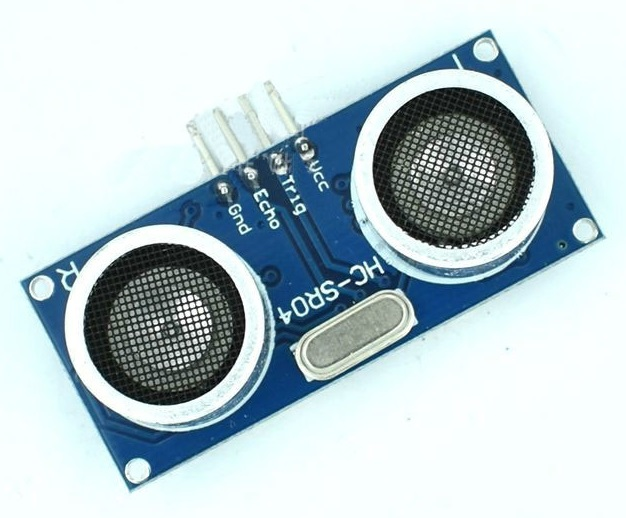在这里简单说下超声波测距的原理，相信大家也都知道。超声波发射装置发出超声波，它的根据是接收器接到超声波时的时间差，与雷达测距原理相似。 超声波发射器向某一方向发射超声波，在发射时刻的同时开始计时，超声波在空气中传播，途中碰到障碍物就立即返回来，超声波接收器收到反射波就立即停止计时。
采用Trig引脚触发，给至少10us的高电平脉冲信号模块自动发送8个40kHz的方波，自动检测是否有信号返回有信号返回，通过Echo引脚输出一个高电平脉冲，高电平脉冲持续的时间就是超声波从发射到反射返回的时间。距离=(高电平脉冲时间*340)/2。（声音在空气中传播速度为340m/s）
主要技术参数工作电压DC5V静态电流<2mA输出电平0-5V感应角度≤15度探测距离2cm-450cm最高精度0.3cm
###舵机   在这里推荐9G舵机SG90，或者其他类似的舵机，这种舵机体积比较小，扭矩虽然不是大， 但是足够带动简易云台，很方便在小车上使用，大家购买时注意舵机的转动角度，有55度的，180度的等等，大家可以根据需要购买。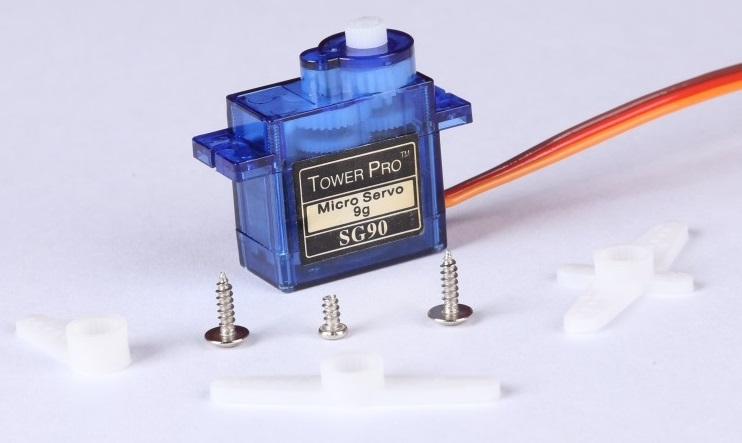舵机固定架
舵机固定架的购买一定要配合舵机，所以大家购买的时候注意尺寸哦！！~

舵机安装
舵机在安装之前大家一定要记得校准，为什么要校准那，这个跟舵机的工作原理有关。控制信号由接收机的通道进入信号调制芯片，获得直流偏置电压。它内部有一个基准电路，产生周期为20ms，宽度为1.5ms的基准信号，将获得的直流偏置电压与电位器的电压比较，获得电压差输出。最后，电压差的正负输出到电机驱动芯片决定电机的正反转。当电机转速一定时，通过级联减速齿轮带动电位器旋转，使得电压差为0，电机停止转动。   舵机的控制一般需要一个20ms左右的时基脉冲，该脉冲的高电平部分一般为0.5ms-2.5ms范围内的角度控制脉冲部分，总间隔为2ms。以180度角度伺服为例，那么对应的控制关系是这样的：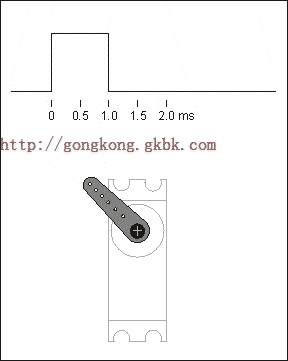高电平时间对应位置0.5ms0度1.0ms45度1.5ms90度2.0ms135度2.5ms180度
也就是说当对舵机输入相同控制信号时，舵机会运动到固定位置，他的动作不是做圆周运动，而是在运动范围内，每一个位置对应一个控制信号。
因此我们需要在将舵机安装在固定架上之前，需要先将舵机初始化好，舵机一般为三根线：棕色——GND，红色——VCC，橙色——控制信号。因此我们将棕色线接到GND，红色线接到“+5V”引脚，橙色线接到“9”引脚，初始化程序如下：
#include <Servo.h>  //加入含有舵机控制库的头文件

#define PIN_SERVO 9  //舵机信号控制引脚
Servo myservo;

void setup()
{
myservo.attach(PIN_SERVO);  //舵机初始化
}

void loop()
{
myservo.write(90);  //PWM输出
}

在舵机初始化好之后将其安装在固定架上，然后装配在小车上，此时保证超声波模块超前。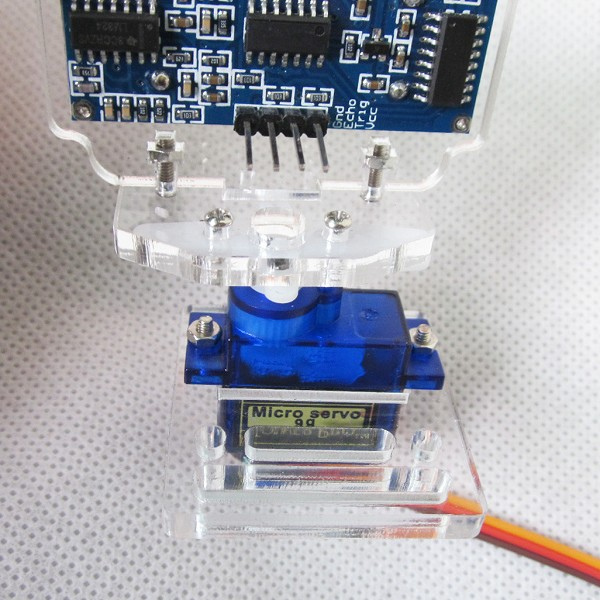超声波接线
估计不少朋友早已经发现板子上的“+5V”和“GND”引脚已经不够用了，这个时候你们可以向我这样焊一个扩展板出来，上面固定两排排针，一排用来扩展“+5V”，一边用来扩展“GND”引脚。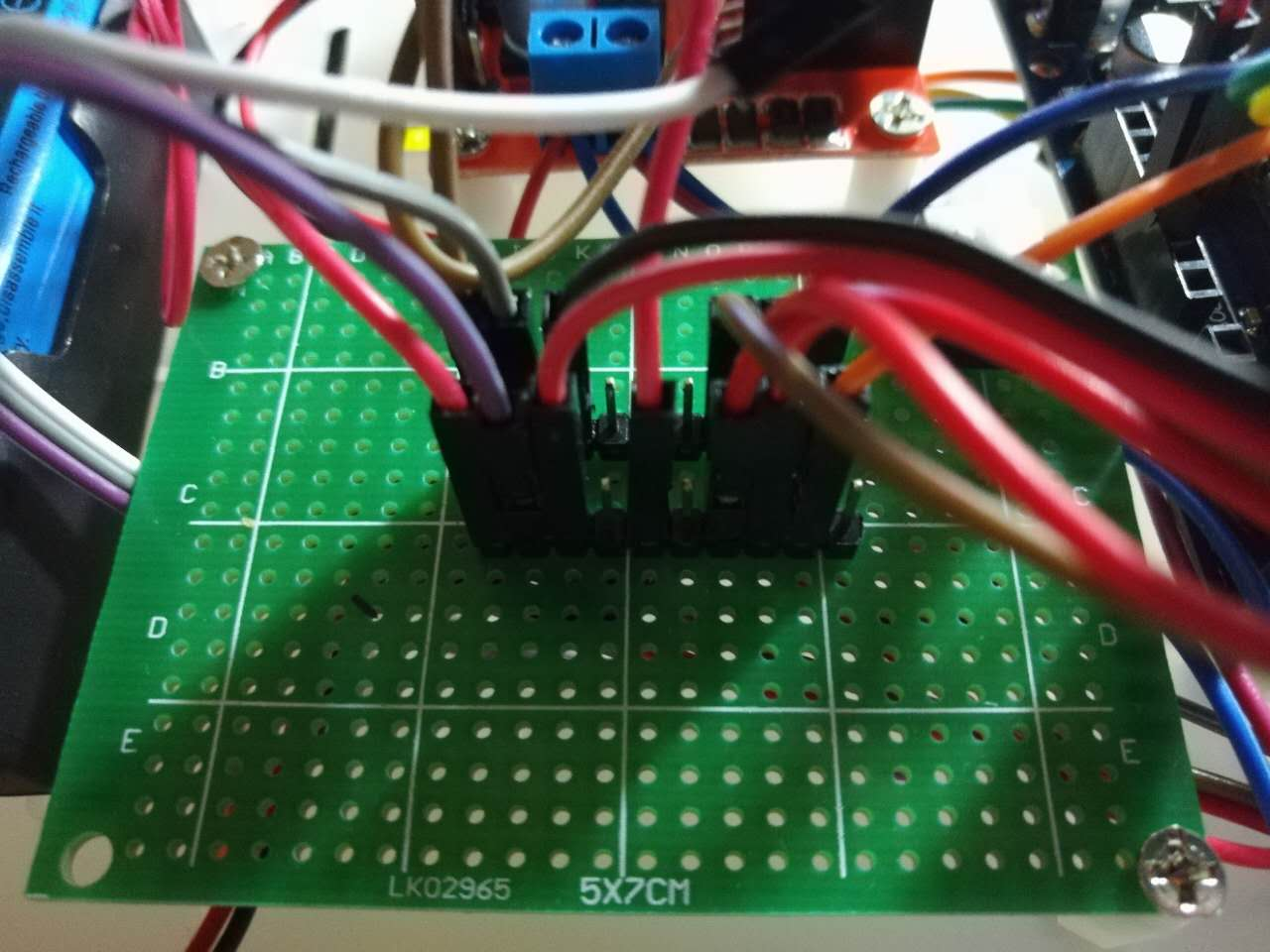超声波模块有四个引脚，“VCC”接到Arduino UNO开发板的“+5V”引脚，“GND”接到开发板“GND”引脚，“Trig”引脚接到开发板“8”引脚，“Echo”引脚接到开发板“7”引脚。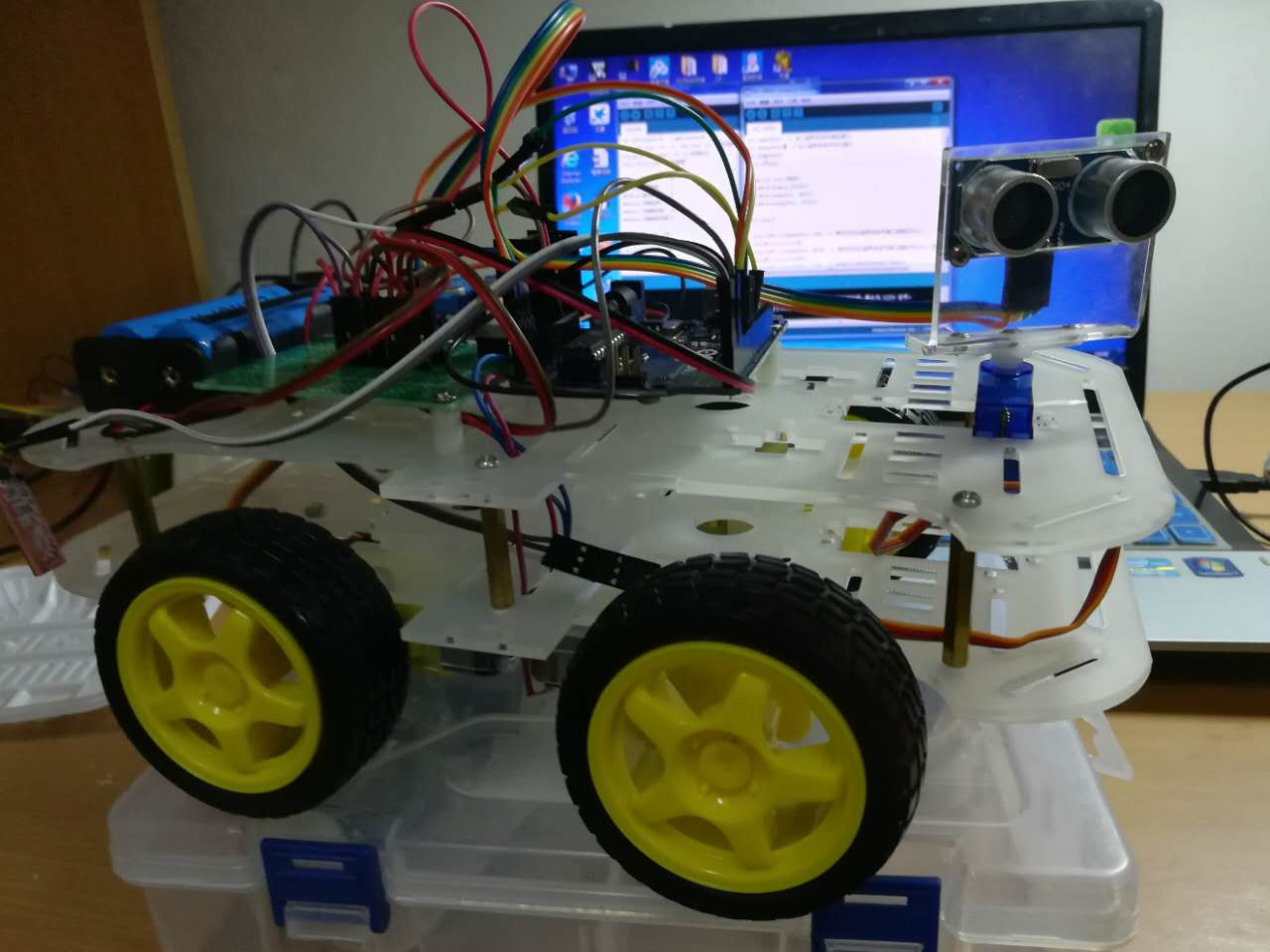线太乱了，真的没办法整理，我自己都没眼看。
代码测试
#include <Servo.h>

#define STOP      0
#define FORWARD   1
#define BACKWARD  2
#define TURNLEFT  3
#define TURNRIGHT 4

int leftMotor1 = 16;
int leftMotor2 = 17;
int rightMotor1 = 18;
int rightMotor2 = 19;

int leftPWM = 5;
int rightPWM = 6;

Servo myServo;  //舵机

int inputPin=7;   // 定义超声波信号接收接口
int outputPin=8;  // 定义超声波信号发出接口

void setup() {
// put your setup code here, to run once:
//串口初始化
Serial.begin(9600);
//舵机引脚初始化
myServo.attach(9);
//测速引脚初始化
pinMode(leftMotor1, OUTPUT);
pinMode(leftMotor2, OUTPUT);
pinMode(rightMotor1, OUTPUT);
pinMode(rightMotor2, OUTPUT);
pinMode(leftPWM, OUTPUT);
pinMode(rightPWM, OUTPUT);
//超声波控制引脚初始化
pinMode(inputPin, INPUT);
pinMode(outputPin, OUTPUT);
}

void loop() {
// put your main code here, to run repeatedly:
avoidance();
}
void motorRun(int cmd,int value)
{
analogWrite(leftPWM, value);  //设置PWM输出，即设置速度
analogWrite(rightPWM, value);
switch(cmd){
case FORWARD:
Serial.println("FORWARD"); //输出状态
digitalWrite(leftMotor1, HIGH);
digitalWrite(leftMotor2, LOW);
digitalWrite(rightMotor1, HIGH);
digitalWrite(rightMotor2, LOW);
break;
case BACKWARD:
Serial.println("BACKWARD"); //输出状态
digitalWrite(leftMotor1, LOW);
digitalWrite(leftMotor2, HIGH);
digitalWrite(rightMotor1, LOW);
digitalWrite(rightMotor2, HIGH);
break;
case TURNLEFT:
Serial.println("TURN  LEFT"); //输出状态
digitalWrite(leftMotor1, HIGH);
digitalWrite(leftMotor2, LOW);
digitalWrite(rightMotor1, LOW);
digitalWrite(rightMotor2, HIGH);
break;
case TURNRIGHT:
Serial.println("TURN  RIGHT"); //输出状态
digitalWrite(leftMotor1, LOW);
digitalWrite(leftMotor2, HIGH);
digitalWrite(rightMotor1, HIGH);
digitalWrite(rightMotor2, LOW);
break;
default:
Serial.println("STOP"); //输出状态
digitalWrite(leftMotor1, LOW);
digitalWrite(leftMotor2, LOW);
digitalWrite(rightMotor1, LOW);
digitalWrite(rightMotor2, LOW);
}
}
void avoidance()
{
int pos;
int dis;//距离
motorRun(FORWARD,200);
myServo.write(90);
dis=getDistance(); //中间

if(dis<30)
{
motorRun(STOP,0);
for (pos = 90; pos <= 150; pos += 1)
{
myServo.write(pos);              // tell servo to go to position in variable 'pos'
delay(15);                       // waits 15ms for the servo to reach the position
}
dis=getDistance(); //左边
for (pos = 150; pos >= 30; pos -= 1)
{
myServo.write(pos);              // tell servo to go to position in variable 'pos'
delay(15);                       // waits 15ms for the servo to reach the position
if(pos==90)
dis=getDistance(); //中间
}
dis=getDistance();  //右边
for (pos = 30; pos <= 90; pos += 1)
{
myServo.write(pos);              // tell servo to go to position in variable 'pos'
delay(15);                       // waits 15ms for the servo to reach the position
}
if(dis<dis) //右边距离障碍的距离比左边近
{
//左转
motorRun(TURNLEFT,250);
delay(500);
}
else  //右边距离障碍的距离比左边远
{
//右转
motorRun(TURNRIGHT,250);
delay(500);
}
}
}
int getDistance()
{
digitalWrite(outputPin, LOW); // 使发出发出超声波信号接口低电平2μs
delayMicroseconds(2);
digitalWrite(outputPin, HIGH); // 使发出发出超声波信号接口高电平10μs，这里是至少10μs
delayMicroseconds(10);
digitalWrite(outputPin, LOW); // 保持发出超声波信号接口低电平
int distance = pulseIn(inputPin, HIGH); // 读出脉冲时间
distance= distance/58; // 将脉冲时间转化为距离（单位：厘米）
Serial.println(distance); //输出距离值

if (distance >=50)
{
//如果距离小于50厘米返回数据
return 50;
}//如果距离小于50厘米小灯熄灭
else
return distance;
}

代码详解
“Trig”引脚控制超声波发出声波，对应int outputPin=8; “Echo”引脚反应接收到返回声波，对应int inputPin=7;
int inputPin=7;   // 定义超声波信号接收接口
int outputPin=8;  // 定义超声波信号发出接口

int getDistance()函数解析
超声波发出引脚“Trig”为高时对外发出超声波，为保证发出10μs声波，因此在发送之前需要将该引脚拉低，并给他一定反应时间。
digitalWrite(outputPin, LOW); // 使发出发出超声波信号接口低电平2μs
delayMicroseconds(2);

之后发送10μs超声波
digitalWrite(outputPin, HIGH); // 使发出发出超声波信号接口高电平10μs，这里是至少10μs

声波发送之后禁止其继续发送，同时开始检测是否反射回来的声波
digitalWrite(outputPin, LOW); // 保持发出超声波信号接口低电平
int distance = pulseIn(inputPin, HIGH); // 读出脉冲时间

pulseIn()单位为微秒，声速344m/s，所以距离cm=344100/1000000pulseIn()/2约等于pulseIn()/58.0distance= distance/58; // 将脉冲时间转化为距离（单位：厘米） 超声波模块工作受物体表面反射程度影响，并且在传播过程中信号强度容易衰减，因此该模块适用的检测距离有限，一般在50cm以内相对正确，而且我们在避障时不需要检测太远的距离，因此超过50cm以上的都按50cm计算
if (distance >=50)
{
//如果距离小于50厘米返回数据
return 50;
}//如果距离小于50厘米小灯熄灭
else
return distance;

void avoidance()函数解析
小车前进过程中只检测前方距离障碍的距离，并且控制舵机，保持超声波模块位于正前方。
motorRun(FORWARD,200);
myServo.write(90);
dis=getDistance(); //中间

当检测到小车前方距离障碍距离小于30cm时停车，检测两边距离。
motorRun(STOP,0);

控制舵机每次运动一个周期后都返回正前方位置。由于舵机运动需要一定的时间，因此在每转过一个角度的时候都延时delay(15)，供其运动。
for (pos = 90; pos <= 150; pos += 1)
{
myServo.write(pos);              // tell servo to go to position in variable 'pos'
delay(15);                       // waits 15ms for the servo to reach the position
}

当运动到最左边时检测小车左边距离障碍的距离
dis=getDistance(); //左边

向右边运动，检测右边距离
for (pos = 150; pos >= 30; pos -= 1)
{
myServo.write(pos);              // tell servo to go to position in variable 'pos'
delay(15);                       // waits 15ms for the servo to reach the position
if(pos==90)
dis=getDistance(); //中间
}
dis=getDistance();  //右边

将前边、左边、右边距离障碍的距离都检测结束之后，舵机回到最初位置。
for (pos = 30; pos <= 90; pos += 1)
{
myServo.write(pos);              // tell servo to go to position in variable 'pos'
delay(15);                       // waits 15ms for the servo to reach the position
}

注意事项
1.舵机使用时要防止其堵转，因为点击堵转时电流会增大，很容易烧坏舵机。 2.舵机的红色电源线接入电压一般要大于等于其工作电压，供电不足会导致舵机不停自传。 3.Arduino 《Servo.h》库里提供的write()函数输出的PWM即为舵机专用的20ms为周期的PWM波，如果使用其他开发板或者函数的话，请务必保证输出方波周期为20ms，否则舵机不会受控制
总结
这一篇讲解了舵机和超声波模块的使用方法，舵机在大家以后的开发生涯中应该会经常用到，因此舵机的使用规则(控制周期为20ms)请大家一定要记清楚，在舵机不受控制的时候建议大家可以购买一个舵机测试仪来测试舵机。

欢迎各位有兴趣的朋友加入Q群1：789127261 点评、交流
展开全文arduino hc-sr04
• 基于单片开机的超声波避障小车，能实现自动避障，显示行驶过的路程 源程序
• 基于STC12单片机系列的智能小车程序，功能能实现超声波测距，识别障碍，主动避障功能，同时还可以通过舵机控制实现对周围环境不同方向的测距功能，可达到正负180°方向识别！
• 超声波避障程序随处可见，基于51单片机的超声波避障小车也很成熟，但是完整的Proteus仿真并不容易找到开源资料。这次主要给大家分享其Proteus仿真部分。涉及到的模块有：超声波模块（hc-sr04）、L293D电机驱动器和...
• 以STM32f103为控制芯片的超声波避障小车程序，带舵机，keil打开编译后即可运行，简单明了。。。。
• stm32 超声波避障小车2stm32 超声波避障小车2stm32 超声波避障小车2stm32 超声波避障小车2
• stm32 超声波避障小车1stm32 超声波避障小车1stm32 超声波避障小车1
• cs-程序\避障\基于80C51单片机小车超声波避障技术设计
• 资源包括：程序程序设计报告 ...思路：通过驱动超声波模块，测量出小车与障碍物之间的距离，并通过蓝牙反馈数据到手机，同时根据测量数据进行舵机的转动控制，从而实现智能避障（建议先看程序设计报告总结部分）...